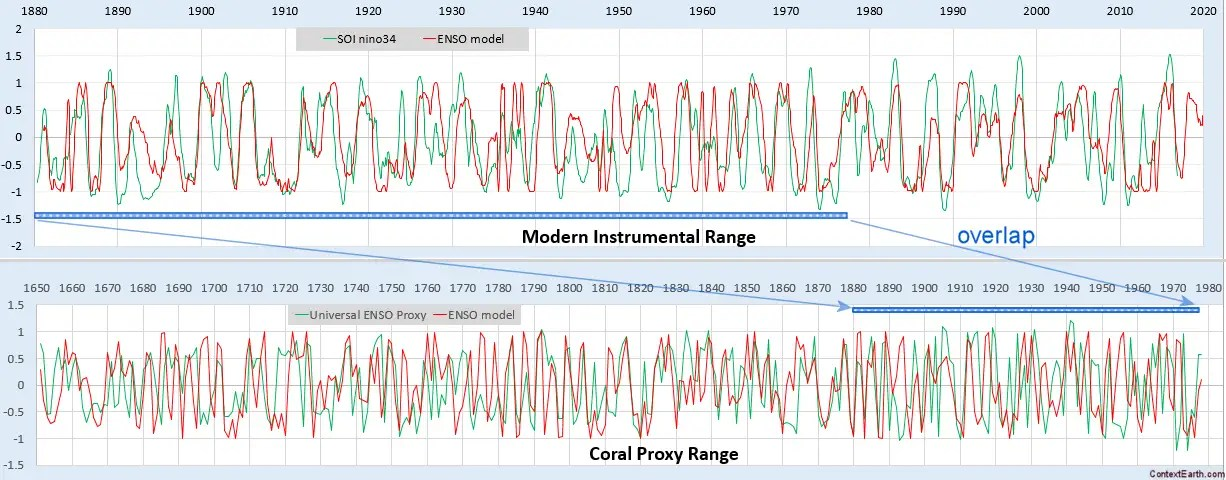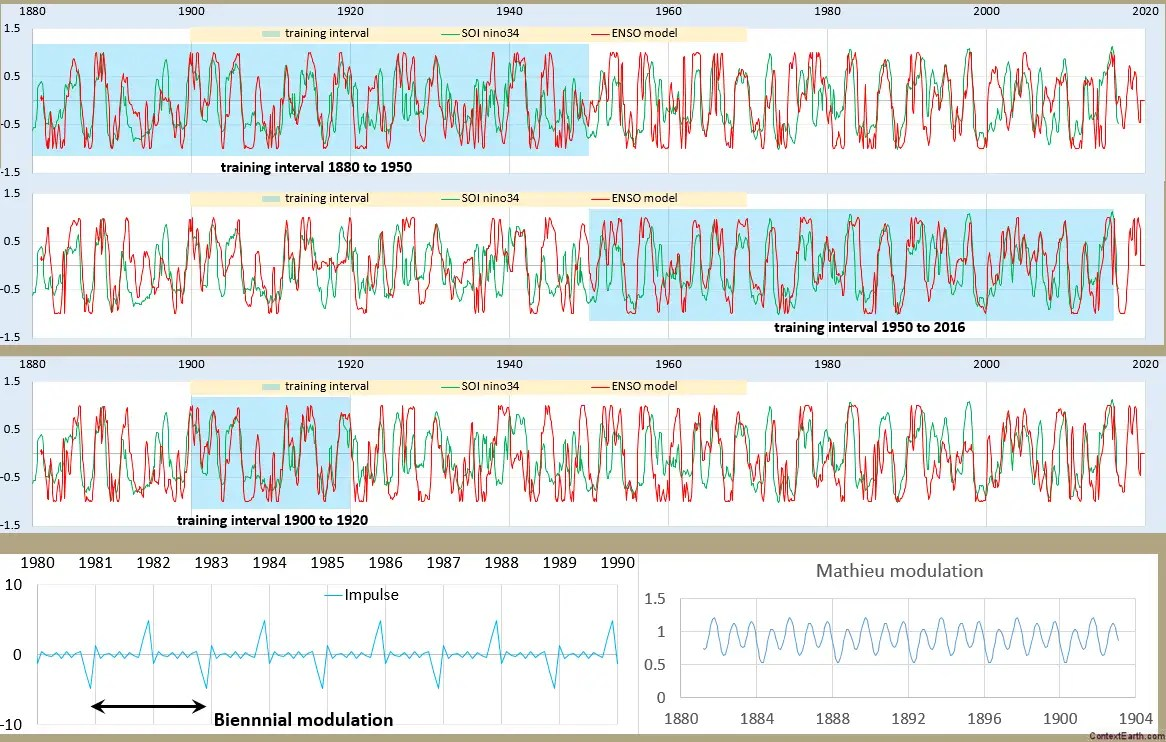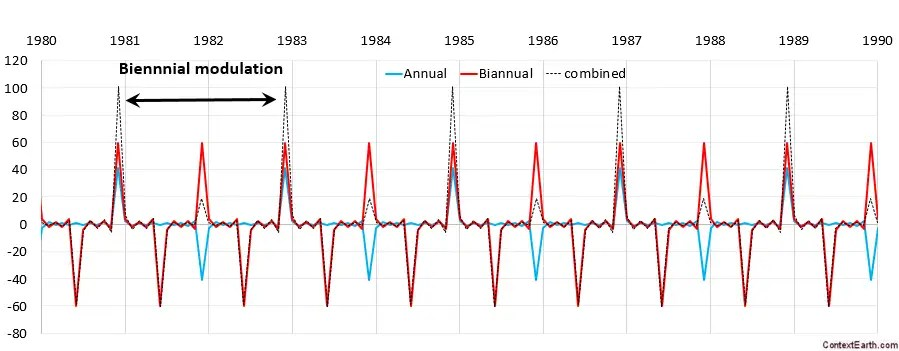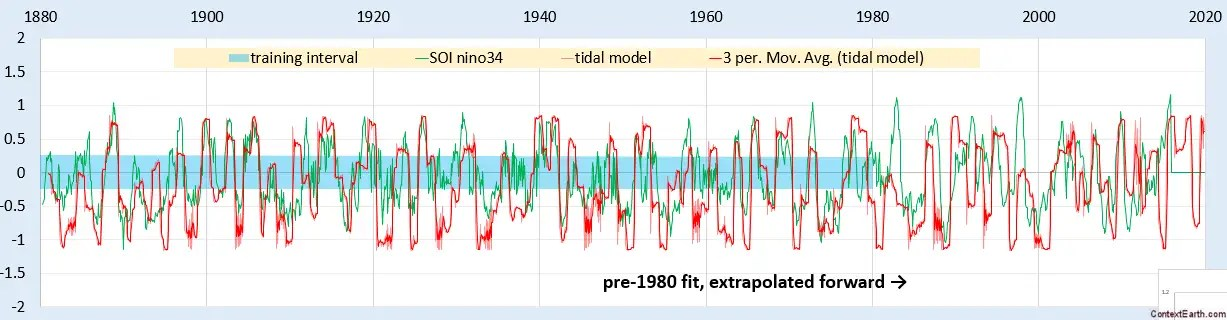# ENSO Proxy Validation

This is a straightforward validation of the ENSO model presented at last December’s AGU.

What I did was use the modern instrumental record of ENSO — the NINO34 data set — as a training interval, and then tested across the historical coral proxy record — the UEP data set.The correlation coefficient in the out-of-band region of 1650 to 1880 is excellent, considering that only two RHS lunar periods (draconic and anomalistic month) are used for forcing. As a matter of fact, trying to get any kind of agreement with the UEP using an arbitrary set of sine waves is problematic as the time-series appears nearly chaotic and thus requires may Fourier components to fit. With the ENSO model in place, the alignment with the data is automatic. It predicts the strong El Nino in 1877-1878 and then nearly everything before that.

# Canonical Solution of Mathieu Equation for ENSO

[mathjax]
From a previous post, we were exploring possible solutions to the Mathieu equation given a pulsed stimulus.  This is a more straightforward decomposition of the differential equation using a spreadsheet.

The Mathieu equation:$f''(t) + omega_0^2 (1 + alpha cos(nu t)) f(t) = F(t)$

can be approximated as a difference equation, where the second derivative f”(t) is ~ (f(t)-2f(t-dt)+f(t-2dt))/dt. But perhaps what we really want is a difference to the previous year and determine if that is enough to reinforce the biennial modulation that we are seeing in the ENSO behavior.

Setting up a spreadsheet with a lag term and a 1-year-prior feedback term, we apply both the biennial impulse-modulated lunar forcing stimulus and a yearly-modulated Mathieu term.Fig. 1: Training (in shaded blue) and test for different intervals.

I was surprised by how remarkable the approximate fit was in the recent post, but this more canonical analysis is even more telling. The number of degrees of freedom in the dozen lunar amplitude terms apparently has no impact on over-fitting, even on the shortest interval in the third chart. There is noise in the ENSO data no doubt, but that noise seems to be secondary considering how the fit seems to mostly capture the real signal. The first two charts are complementary in that regard — the fit is arguably better in each of the training intervals yet the test interval results aren’t really that much different from the direct fit looking at it by eye.

Just like in ocean tidal analysis, the strongest tidal cycles dominate;  in this case the Draconic and Anomalistic monthly, the Draconic and Anomalistic fortnightly, and a Draconic monthly+Anomalistic fortnightly cross term are the strongest (described here). Even though there is much room for weighting these factors differently on orthogonal intervals, the Excel Solver fit hones in on nearly the same weighted set on each interval.  As I said in a previous post, the number of degrees of freedom apparently do not lead to over-fitting issues.

One other feature of this fit was an application of a sin() function applied to the result. This is derived from the Sturm-Liouville solution to Laplace’s tidal equation used in the QBO analysis  — which works effectively to normalize the model to the data, since the correlation coefficient optimizing metric does not scale the result automatically.

Pondering for a moment, perhaps the calculus is not so different to work out after all:

A bottom-line finding is that there is really not much complexity to this unique tidal formulation model of ENSO.  But because of the uniqueness of the seasonal modulation that we apply, it just doesn’t look like the more-or-less regular cycles contributing to sea-level height tidal data.  Essentially similar algorithms are applied to find the right weighting of tidal factors, but whereas the SLH tidal data shows up in daily readings, the ENSO data is year-to-year.

Further, the algorithm does not take more than a minute or two to finish fitting the model to the data. Below is a time lapse of one such trial. Although this isn’t an optimal fit, one can see how the training interval solver adjustment (in the shaded region) pulls the rest of the modeled time-series into alignment with the out-of-band test interval data.# Tidal Model of ENSO

The input forcing to the ENSO model includes combinations of the three major lunar months modulated by the seasonal solar cycle. This makes it conceptually similar to an ocean tidal analysis, but for ENSO we are more concerned about the long-period tides rather than the diurnal and semi-diurnal cycles used in conventional tidal analysis.

The three constituent lunar month factors are:

Month type Length in days
anomalistic 27.554549
tropical 27.321582
draconic 27.212220

So the essential cyclic terms are the following phased sinusoids

# Solution to Pulsed Mathieu Equation

Here is a bit of applied math that I have never seen described before.  It considers solving a variant of the Mathieu differential equation, an unwieldy beast that finds application in models of fluid sloshing (among others). Normally the Mathieu equation is only solvable as a stimulus forcing function convolved against the transcendental Mathieu function. Tools such as Mathematica include the Mathieu function in their library, but any other solution would require a full numerical DiffEq integration.

This is the typical Mathieu equation formulation:$f''(t) + ( omega_0^2 + B cos (nu_0 t) ) f(t) = 0$

But instead of this nonlinear time-varying DiffEq, consider that we replace the sinusoidal modulation with a delta pulse. This is precisely our model of ENSO that we are trying to create from first principles. The pulse represents a forcing impulse from the alignment of a lunar and solar tidal cycle.$f''(t) + ( omega_0^2 + B delta (t) ) f(t) = 0$

Taking the Laplace transform, we quickly arrive at what looks like an ordinary 2nd-order differential equation solution, albeit with an interesting initial condition:$s^2 F(s) + omega_0^2 F(s) + B f(0) = 0$

After taking the inverse Laplace transform, we have:$f(t) = I(t) ast B f(0) delta(t)$

where I(t) represents the impulse response of the first two terms, which is then convolved with a delta function evaluated at a value of f(t) for t=0. This is the only remnant of the nonlinear nature of the classical Mathieu function, in that the initial condition has a scaling proportional to the value of the function at that time. On the other hand, the solution to an ordinary DiffEq would not be dependent on the value of the function.

Thereafter we can extend this to a general solution; by creating a pulse train of delta functions we get this final convolution:$f(t) = I(t) ast B sum_{n=0}^{infty} f(t - n T) delta(t - n T)$

where T is the pulse train period.

This is straightforward to evaluate for any pulse train — all we have to do is keep track of the changing value of f(t) as we come across each pulse.Fig 1: A pulse train with both annual and biannual contributions.

In the above figure, why does the annual pulse have a two-year periodicity? That’s due to the alignment of a non-congruent tidal period with a seasonal pulse in terms of a Fourier series as described here and mathematically refined here.

If that is not intuitive, we can still consider it more of an anzat and see where it takes us.

For an ENSO time series, we an convolve the above delta pulse train with a set of known tidal periods of arbitrary amplitude and phase. For a fit trained up to 1980, this is the extrapolation post-1980:Fig 2: Fit of pulsed Mathieu equation using a set of tidal periods as a forcing function. The impulse response here is a simple year-long rectangular window, i.e. the response has a memory of only a calendar year. This can be further refined if necessary. Yet, the projected time-series evaluated out-of-band from the fitting interval does a good job of capturing the ENSO profile.

The projected waveform matches the last 16 years very effectively considering how noisy the ENSO series is, and is very close to the fit over the entire interval. This is apart from the possible perturbation around the 1982 El Nino. The fact that the monthly tidal periods differ by slight amounts dictates that long multi-decadal intervals should be used for fitting. (In contrast, the model of QBO is primarily Draconic so the fitting interval can be much shorter)Fig 3: Fit over the entire interval. The set of tidal periods applied was limited to the 3 major months (draconic, anomalistic, and sidereal/tropical) and the multiplicative combinations creating the fortnightly tides. This may not be enough to get the details right but erred on the side of under-fitting to establish the physical mechanism.

This approach is the logical follow-on to the wave transformed fitting approach that I had been using, most recently here and here. Both of these approaches are equally clever, which is a necessary ingredient when one is dealing with the unwieldy Mathieu equations. Moreover they both pull out the obvious stationary aspects of the ergodic ENSO time series.

# The ENSO Challenge

It’s been quite a challenge decoding the physics of ENSO. Anything that makes the model more complex and with more degrees of freedom needs to be treated carefully. The period doubling bifurcation properties of wave sloshing has been an eye-opener for me. I experimented with adding a sub-harmonic period of 4 years to the 2-year Mathieu modulation and see if that improves the fit. By simply masking the odd behavior around 1981-1983, I came up with this breakdown of the RHS/LHS comparison.

# Solver vs Multiple Linear Regression for ENSO

In the previous ENSO post I referenced the Rajchenbach article on Faraday waves.

There is a telling assertion within that article:

“For instance, to the best of our knowledge, the dispersion relation (relating angular frequency ω and wavenumber k) of parametrically forced water waves has astonishingly not been explicitly established hitherto. Indeed, this relation is often improperly identified with that of free unforced surface waves, despite experimental evidence showing significant deviations”

What they are suggesting is that too much focus has been placed on natural resonances and the dispersion relationships within a free fluid volume. Whereas the forced response is clearly as important — if not more — and that the forcing will show through in the solution of the equations. I have been pursuing this strategy for a while, having started down the Mathieu equation right away and then eventually realizing the importance of the forced response, yet the Rajchenbach article is the first case that I have found made of what I always thought should be a rather obvious assumption. The fact that the peer-reviewers allowed the “astonishingly” adjective in the paper is what makes it telling. It’s astonishing in the equivalent sense that Rajchenbach & Clamond are pointing out that a pendulum’s motion will be impacted by a periodic push. In other words, astonishing in the sense that this premise should be obvious!

# Short Training Intervals for QBO

[mathjax]An old game show called Name That Tune asked contestants to identify a song by listening to just a few notes.

This post is the QBO equivalent to that. How short an interval can we train on to reproduce the rest of the time series? The answer is not much is required.

# Modeling Red Noise versus ENSO

With respect to the ENSO model I have been thinking about ways of evaluating the statistical significance of the fit to the data. If we train on one 70 year interval and then test on the following 70 year interval, we get the interesting effect of finding a higher correlation coefficient on the test interval. The training interval is just below 0.85 while the test is above 0.86.This image has been resized to fit in the page. Click to enlarge.

# ENSO Proxy Revisited

Although the historical coral proxy measurements are not high resolution (1 year resolution available), they can provide substantiation for models of the modern day instrumental record. This post is a revisit of a previous analysis of the Universal ENSO Proxy (UEP).

The interval from 1881 to 1950 of the ENSO data was used to train the DiffEq ENSO model. This gives a higher correlation coefficient (~0.85) on the test interval (from 1950 to 2014) than the training interval (1881-1950) as shown below:Fig. 1: ENSO model fit over the modern instrumental record

Since ENSO data shows stationarity and coherence over the interval of 70 years, this fit was re-applied to the UEP data over 4 different ranges 1650-1720, 1720-1790, 1790-1860, and 1860-1930. High correlation coefficients were found for each of these intervals (> 0.70) and compared against fits to a red noise model shown below:Fig 2: Proxy fits over various ranges as compared to a red noise model (added for clarification: essentially a synthetic data set to evaluate the ENSO model against). The significance is at least at 0.95 for each proxy interval.

Each of the ENSO fits lies within the 0.95 significance level, and only 1 out of 500 red noise simulations obtained a 0.8 correlation coefficient, which is what the 1720-1790 interval achieved.

Interval CC
1650-1720 0.772
1720-1790 0.807
1790-1860 0.710
1860-1930 0.763

The significance of having each of these intervals at least 0.95 is 1-(1-0.95)^4 if these are all independent. That is a small number less than about (1/20)^4 in likelihood.

The caveat is that the ENSO is also not likely to be coherent over intervals much greater than 70 years, as the shift around 1980 in phase for the model demonstrates.

This substatntiates the finding of Hanson, Brier, Maul  that ENSO and El Nino frequencies may be relatively constant back to the year 1525.

Astudillo et al  also confirmed the ENSO shift after 1980 stating that “the amplitude of the 1982-1983 event is unique”.  Further they concisely describe the ENSO behavior as being highly deterministic by stating:

“This is of crucial importance since if a system is deterministic, the vector field at every period of the state space is uniquely defined by a set of ordinary differential equations.”

## References

 K. Hanson, G. W. Brier, and G. A. Maul, “Evidence of significant nonrandom behavior in the recurrence of strong El Niño between 1525 and 1988,” Geophysical Research Letters, vol. 16, no. 10, pp. 1181–1184, 1989.

 H. Astudillo, R. Abarca-del-Río, and F. Borotto, “Long-term potential nonlinear predictability of El Niño–La Niña events,” Climate Dynamics, pp. 1–11, 2016.